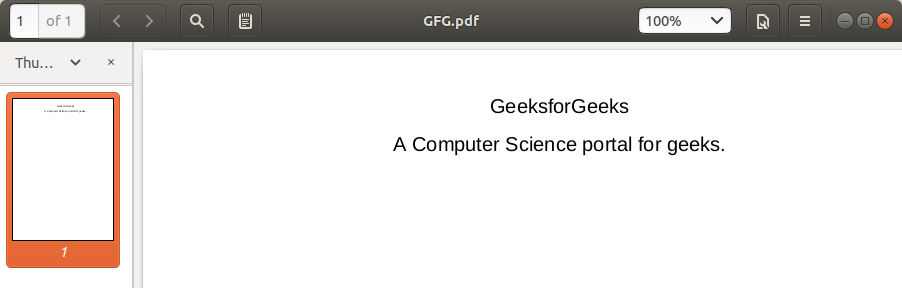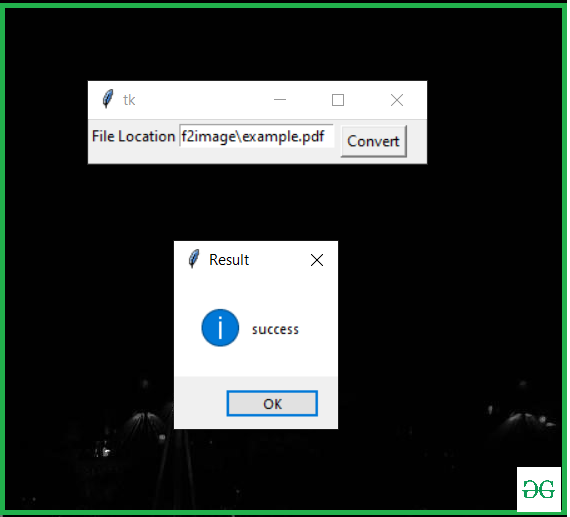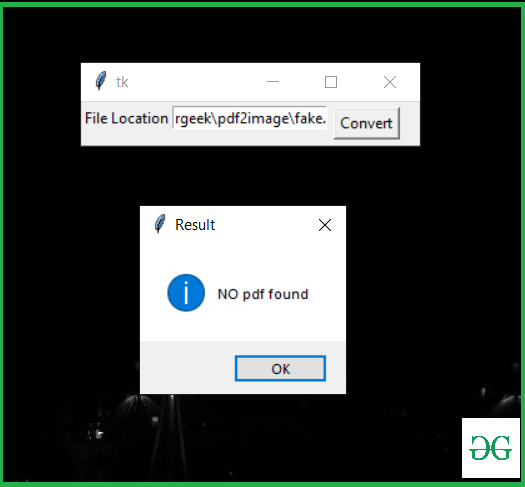# Convert PDF to Image using Python

• Difficulty Level : Easy
• Last Updated : 21 Jan, 2021

Many tools are available on the internet for converting a PDF to an image. In this article, we are going to write code for converting pdf to image and make a handy application in python. Before writing the code we need to install the required module pdf2image and poppler.

### Modules Needed

• pdf2image 1.14.0: This module converts a PDF to a PIL object. To install this module type the below command in the terminal.
pip install pdf2image

You will then have to add the bin/ folder to PATH or use

poppler_path = r”C:\path\to\poppler-xx\bin” as an argument in convert_from_path.

Approach:

• Import the pdf2image module
• Store a PFD with convert_from_path()
• Save image with save()

Below is the Implementation.

PDF File used:## Python

 # import modulefrom pdf2image import convert_from_path  # Store Pdf with convert_from_path functionimages = convert_from_path('example.pdf') for i in range(len(images)):         # Save pages as images in the pdf    images[i].save('page'+ str(i) +'.jpg', 'JPEG')

Output:Let’s write code for Application Using Tkinter: This Script implements the above Implementation into a GUI.

Below is the Implementation.

## Python3

 from pdf2image import convert_from_pathfrom tkinter import *from tkinter import messagebox  def pdf2img():    try:        images = convert_from_path(str(e1.get()))        for img in images:            img.save('new_folder\output.jpg', 'JPEG')     except  :        Result = "NO pdf found"        messagebox.showinfo("Result", Result)     else:        Result = "success"        messagebox.showinfo("Result", Result)   master = Tk()Label(master, text="File Location").grid(row=0, sticky=W) e1 = Entry(master)e1.grid(row=0, column=1) b = Button(master, text="Convert", command=pdf2img)b.grid(row=0, column=2,columnspan=2, rowspan=2,padx=5, pady=5)  mainloop()

Output:If there is no PDF file at your given location.My Personal Notes arrow_drop_up